﻿ 基于机器学习的雷达回波与降雨分析

# 基于机器学习的雷达回波与降雨分析Relationship Analysis of Radar Echo and Rainfall Based on Machine Learning

Abstract: Radar echo data generated by Doppler weather radar is an important basis for rainfall analysis and prediction. Aiming at the problem of how to make effective use of radar echo for rainfall grade analysis, this paper studies an analysis model of the relationship between radar echo and rainfall based on XGBoost ensemble learning algorithm. In this paper, we use the radar and rainfall mete-orological observation data provided by Liaoning Meteorological Station over the years. After data decoding, cleaning and matching, we use XGBoost method optimized by grid search algorithm to establish the classification relationship between multi-layer radar echo data and rainfall level. Fi-nally, the experimental results show that the results based on XGBoost method are closer to reality and can better reflect the relationship between cloud radar echo and rainfall.

1. 引言

2. 多层雷达数据与降雨关系分析

2.1. 雷达和降雨数据集的处理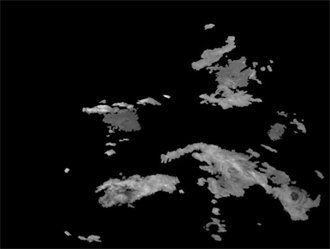Figure 1. Visualize radar map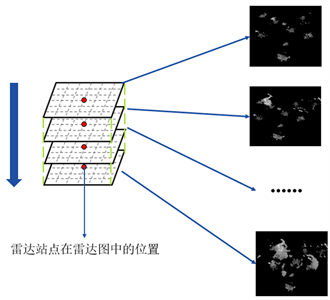Figure 2. Multilayer radar data organization form

$dBZ=\frac{\left(Grey-66\right)}{2}$ (1)

2.2. 基于XGBoost的雷达回波与降雨分析系统

2.2.1. XGBoost集成学习算法

XGBoost是GBDT (Gradient Boosting Decision Tree)的改进版本。为了避免过拟合问题，它将树模型的复杂程度纳入到正则项中；损失函数使用泰勒展开式展开，使用了一阶导数与二阶导数。经历 次迭代后样本i的分析结果可以被表示为：前 棵棵决策树分析结果与使用迭代函数 的第 次迭代结果之和。

${\stackrel{^}{y}}_{i}^{\left(t\right)}=\underset{k=1}{\overset{t}{\sum }}{f}_{k}\left({x}_{i}\right)={\stackrel{^}{y}}_{i}^{\left(t-1\right)}+{f}_{t}\left({x}_{i}\right)$ (2)

XGBoost的目标函数可被表示为式(3)，其中，第一项为损函数差，例如常见的logistic或MSE，其代表了模型的偏差；为了尽可能减小模型方差，控制树的复杂程度，在一定程度上防止发生过拟合现象 ，在目标函数中加入了第二项正则项 $\Omega$，它的更详细表示见式(4)。式(4)中，T表示每棵树叶子节点的数量，其值越小表示模型越简单， $\omega$ 表示这些树叶子节点权重组成的集合， $\omega$ 中权重不宜过高， $\gamma$$\lambda$ 是参数，可依据实际应用自行设置。

$L\left(\phi \right)=\underset{i}{\sum }l\left({\stackrel{^}{y}}_{i},{y}_{i}\right)+\underset{k}{\sum }\Omega \left({f}_{k}\right)$ (3)

$\Omega \left(f\right)=\gamma T+\frac{1}{2}\lambda {‖\omega ‖}^{2}$ (4)

2.2.2. 基于XGBoost的雷达回波与降雨分析模型优化

K折交叉验证(K-fold cross validation)是一种检验分类器性能的统计学方法 。其基本思想是将训练集分为K份(均等划分，K ≥ 2)，其中K − 1份作为训练集，带入模型训练，剩余1份子集作为验证集 。上述过程重复K次，验证集每次选取第1，2，3，……，K份子集，记录每次验证集得分Ei(1 < i ≤ K)，并求得均值作为得分 。网格搜索与交叉验证关系见图3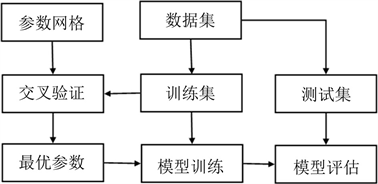Figure 3. Grid Search and Cross Validation

2.2.3 降水分析系统的设计与实现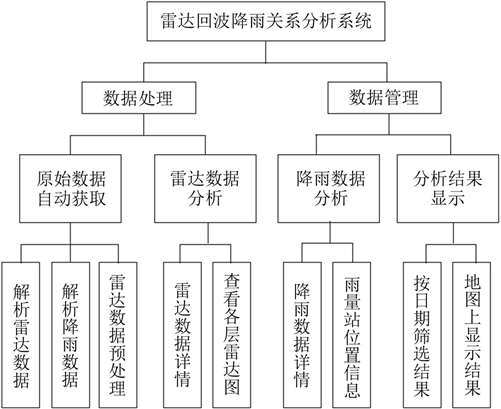Figure 4. System functional block diagram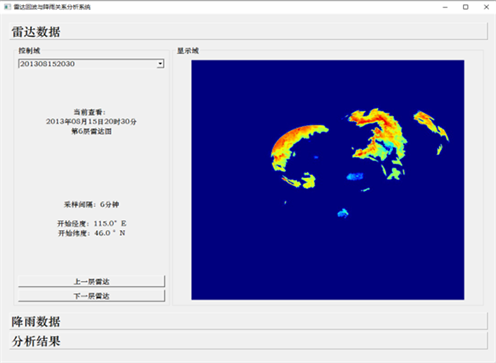Figure 5. Radar data analysis moduleFigure 6. Analysis results display

3. 实验结果及分析Table 1. Abbreviations used in evaluation method

$accuracy=\frac{TP+TN}{TP+TN+FP+FN}$ (5)

$precision=\frac{TP}{TP+FP}$ (6)

$recall=\frac{TP}{TP+FN}$ (7)

${F}_{1}score=\frac{precision×recall×2}{precision+recall}$ (8)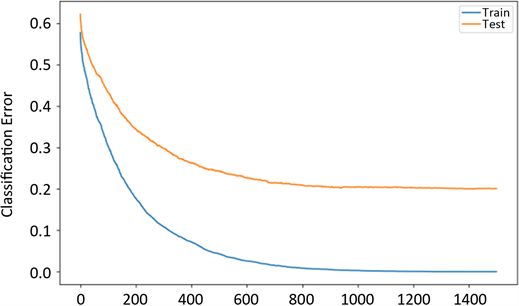Figure 7. Relationship of iteration and error rate system result of standard experimentTable 2. Each classification level index data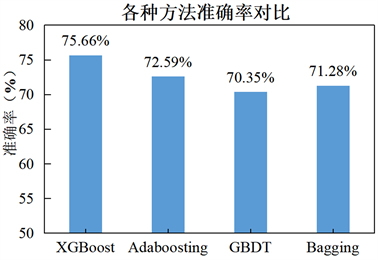Figure 8. The accuracy of various methods

4. 结论

 王慧媛. 基于深度学习的短时定量降水预测研究[D]: [学位论文]. 金华: 浙江师范大学, 2020.

 Suzana, R. and Wardah, T. (2011) Radar Hydrology: New Z/R Relationships for Klang River Basin, Malaysia. Proceedings of 2011 International Conference on Environment Science and Engineering, Bali Island, 1-3 April 2011.

 汪瑛, 冯业荣, 蔡锦辉, 胡胜. 雷达定量降水动态分级Z-I关系估算方法[J]. 热带气象学报, 2011, 27(4): 601-608.
http://dx.chinadoi.cn/10.3969/j.issn.1004-4965.2011.04.018

 Brandes, E.A. (1975) Optimizing Rainfall Es-timates with the Aid of Radar. Journal of Applied Meteorology and Climatology, 14, 1339-1345.
https://doi.org/10.1175/1520-0450(1975)014%3C1339:OREWTA%3E2.0.CO;2

 Sakaino, H. (2013) Spa-tio-Temporal Image Pattern Prediction Method Based on a Physical Model with Time-Varying Optical Flow. IEEE Transactions on Geoscience and Remote Sensing, 51, 3023-3036.
https://doi.org/10.1109/TGRS.2012.2212201

 Lee, S., Cho, S. and Wong, M. (1998) Rainfall Prediction Us-ing Artificial Neural Networks. Journal of Geographic Information and Decision Analysis, 2, 233-242.

 Kufigowski, R.J. and Barros, A.P. (1998) Localized Precipitation Forecasts from a Numerical Weather Prediction Model Using Artificial Neural Networks. Weather and Forecasting, 13, 1194-1204.
https://doi.org/10.1175/1520-0434(1998)013%3C1194:LPFFAN%3E2.0.CO;2

 Luk, K.C., Ball, J.E. and Sharma, A. (2001) An Application of Artificial Neural Networks for Rainfall Forecasting. Mathematical and Computer Modelling, 33, 683-693.
https://doi.org/10.1016/S0895-7177(00)00272-7

 Chau, K.W. and Wu, C.L. (2010) A Hybrid Model Coupled with Singular Spectrum Analysis for Daily Rainfall Prediction. Journal of Hydroinformatics, 12, 458-473.
https://doi.org/10.2166/hydro.2010.032

 时玮域. 基于机器学习方法的雾天气预测研究[D]: [硕士学位论文]. 沈阳: 沈阳工业大学, 2020.

 王训师. XGBoost机器学习模型在缺血性卒中后早期认知损害诊断中的应用研究[D]: [博士学位论文]. 杭州: 浙江大学, 2018.

 王晓晖, 张亮, 李俊清, 孙玉翠, 田捷, 韩睿毅. 基于遗传算法与随机森林的XGBoost改进方法研究[J]. 计算机科学, 2020, 47(Z2): 454-458+463.

 Zhang, X.M., Yan, C., Gao, C., Malin Bradley, A. and Chen, Y. (2020) Predicting Missing Values in Medical Data via XGBoost Regression. Journal of Healthcare Informatics Research, 4, 383-394.
https://doi.org/10.1007/s41666-020-00077-1

 陈逸伦. 基于多源卫星数据的云团和雨团识别及其特征研究[D]: [博士学位论文]. 合肥: 中国科学技术大学, 2019.

 Kato, S., Rose, F.G., Rutan, D.A., Thorsen, T.J., Loeb, N.G., Doelling, D.R., et al. (2018) Surface Irradiances of Edition 4.0 Clouds and the Earth’s Radiant Energy System (CERES) Energy Balanced and Filled (EBAF) Data Product. Journal of Climate, 31, 4501-4527.
https://doi.org/10.1175/JCLI-D-17-0523.1

 Guo, J.Q., Dai, Y.Z., Wang, C.X., Wu, H., Xu, T.Y. and Lin, K. (2020) A Physiological Data-Driven Model for Learners’ Cognitive Load Detection using HRV-PRV Feature Fusion and Optimized XGBoost Classification. Software: Practice and Experience, 50, 2046-2064.
https://doi.org/10.1002/spe.2730

Top